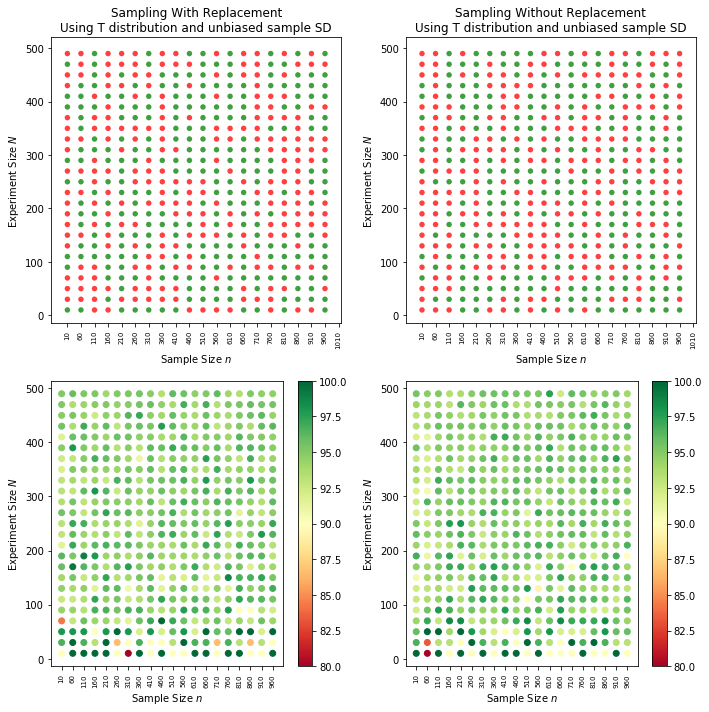Create Population¶

In :
%matplotlib inline

import sys
sys.path.insert(0, '../../')

import matplotlib.pyplot as plt
from SDSPSM import get_metrics, drawBarGraph
from ci_helpers import create_bernoulli_population

T = 4000   # total size of population
p = 0.6    # 60% has yellow balls

# create population
population, population_freq = create_bernoulli_population(T,p)

# population metrics
mu, var, sigma = get_metrics(population)

# visualize
fig, (ax1) = plt.subplots(1,1, figsize=(5,3))
drawBarGraph(population_freq, ax1, [T, mu, var, sigma], 'Population Distribution','Gumballs', 'Counts',xmin=0)
plt.show()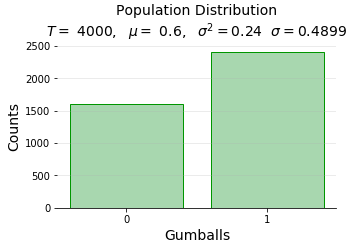Sample and calculate CI along the way¶

In :
import numpy as np
from random import choices
from numpy.random import choice

def sample_wor(population, sample_size):

p = population
ss = sample_size
s = []

if len(p) < ss:
raise ValueError('sample size {} greater than population size {}'.format(ss,len(p)))

for i in range(0,ss):
random_index = np.random.randint(0, len(p))
s.append(p.pop(random_index))

return s,p  # return sample and reduced population

def sample_with_CI(N, n, population, sigma=1, mode='z'):
"""
N - no of trials/experiments
n - sample size
sigma - population SD (needed in z mode)
"""
Y_hat = []
Y_mean_list = []
CI_list = []
for each_experiment in range(N):

#         Y_hat = choices(population, k=n)
Y_hat = choice(population, size=n, replace=False)
Y_mean = sum(Y_hat)/len(Y_hat)

if mode == 'z': # then use population SD sigma, not typically practical
c = 1.96  # which comprises of 95% of data points in std. normal distribution
Y_sigma = sigma
CI_err = round(c*Y_sigma,4)
else:  # t distribution, use unbiased variance
from scipy import stats
c = stats.t.ppf(1-0.025, n-1)  # t value varies depending on degrees of freedom
Y_variance = sum([(y - Y_mean)**2 for y in Y_hat])/(n-1)  # unbiased estimator
Y_sigma = round(sqrt(Y_variance), 4)
CI_err = round(c*(Y_sigma/sqrt(n)),4)

CI_list.append((Y_mean, CI_err))
Y_mean_list.append(Y_mean)

return Y_mean_list, CI_list

.. for sample size 50 and no of experiments 100 (so as to view the CI performance for all 100 sample proportions)

In :
from random import seed
from ci_helpers import mini_plot_SDSP

N = 100
n = 50

#seed(0)

# sample from population
Y_mean_list, CI_list = sample_with_CI(N, n, population, sigma=sigma, mode='z')

# sample metrics
mu, var, sigma = get_metrics(Y_mean_list)

# visualize
fig, (ax1,ax2,ax3) = plt.subplots(1,3,figsize=(15,4))
mini_plot_SDSP(Y_mean_list,ax1,ax2,ax3,width=0.05,  norm_off=True)

from IPython.display import display, Math
display(Math(r'\mu_{{\hat{{p}}}}:{} \ \ \ \ \sigma_{{\hat{{p}}}}:{}'.format(mu, sigma)))
$$\mu_{\hat{p}}:0.5994 \ \ \ \ \sigma_{\hat{p}}:0.0748$$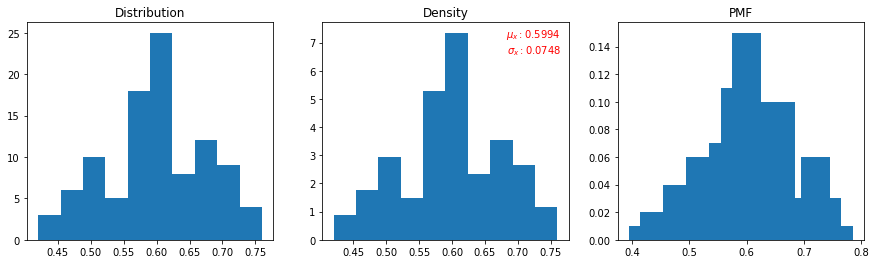Plot CI¶

In :
from ci_helpers import plot_ci_accuracy_1

fig, ax = plt.subplots(1,1, figsize=(20,5))

plot_ci_accuracy_1(ax, CI_list, mu)
plt.show()
CI containing pop.mean:100.0%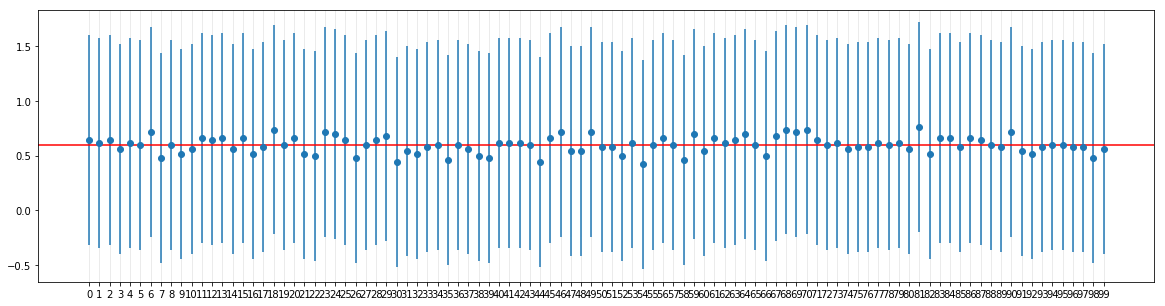Varying N,n, sample with replacement¶

In :
from math import sqrt
from random import sample

def repeated_experiments_with_CI(population,mu, sigma, N_list=[], n_list=[],  mode=1, dist=0, format='b', replace=True):
"""
population - raw population from which sample to be taken
mu, sigma - population parameters
C - 85% CI constant (for eg, 1.96 or 2.093 etc)
N_list - Experiment size or no of times experiments to be conducted per trial
n_list - Sample size or no of samples per experiment
Mode 1 - use population SD
Mode 2 - use unbiased sample SD
Mode 3 - usebiased sample SD
dist - 0 - use Z distribution
dist - 1 - use t distribution
"""
accuracy_list = []
for each_N in N_list:  # no of experiments
for each_n in n_list:   # sample size for each experiment
err_count = 0
for each_E in range(each_N): # for each experiment of experiment size
#                 Y_hat = sample(population, k=each_n)  # pick n samples
Y_hat = choice(population, size=each_n, replace=replace)
Y_mean = sum(Y_hat)/len(Y_hat)

if dist == 0:
C = 1.96
elif dist == 1:
from scipy import stats
C = stats.t.ppf(1-0.025, each_n-1)  # t value varies depending on degrees of freedom  which is (no of samples - 1)

if mode == 1:
Y_sigma = sigma
CI_err = round(C*Y_sigma,4)  # if population SD, there is no sample size in denominator
elif mode == 2:
Y_variance = sum([(y - Y_mean)**2 for y in Y_hat])/(each_n-1)  # unbiased estimator
Y_sigma = round(sqrt(Y_variance), 4)
CI_err = round(C*(Y_sigma/sqrt(each_n)),4)
elif mode == 3:
Y_variance = sum([(y - Y_mean)**2 for y in Y_hat])/(each_n)  # biased estimator
Y_sigma = round(sqrt(Y_variance), 4)
CI_err = round(C*(Y_sigma/sqrt(each_n)),4)
else:
raise ValueError('Wrong mode')
low_err = Y_mean - CI_err
hig_err = Y_mean + CI_err
#print(CI_err,Y_mean,low_err,hig_err,mu)
if (hig_err <= mu) or (low_err >= mu):
err_count += 1
accuracy = round((1-err_count/each_N)*100,4)
success = 0 if accuracy < 95 else 1
if format == 'b':
accuracy_list.append((each_N, each_n, success))
else: # anything other than b
accuracy_list.append((each_N, each_n, accuracy))
return accuracy_list
In :
from ci_helpers import plot_ci_accuracy_2, get_dist_label, get_mode_label

fig, axarr = plt.subplots(2,3, figsize=(15,10))

m , _ , s = get_metrics(population)
dist = 0
max_sample_size = int(T/4)  # 25% of total population
N_list = range(5,100,20)
n_list = range(5,max_sample_size,50)  # different sample sizes

for i in range(1,4): # 1,2,3 modes
for each_N in N_list:
for each_n in n_list:

dist=0  # z dist
ax = axarr[0,i-1]
accuracy_list = repeated_experiments_with_CI(population,m,s,[each_N],[each_n],i,dist,'b', replace=True)
plot_ci_accuracy_2(ax, accuracy_list)
ax.set_title('Using {} and {}'.format(get_dist_label(dist), get_mode_label(i)))

dist=1   # t dist
ax = axarr[1,i-1]
accuracy_list = repeated_experiments_with_CI(population,m,s,[each_N],[each_n],i,dist,'b', replace=True)
plot_ci_accuracy_2(ax, accuracy_list)
ax.set_title('Using {} and {}'.format(get_dist_label(dist), get_mode_label(i)))

plt.tight_layout()
plt.show()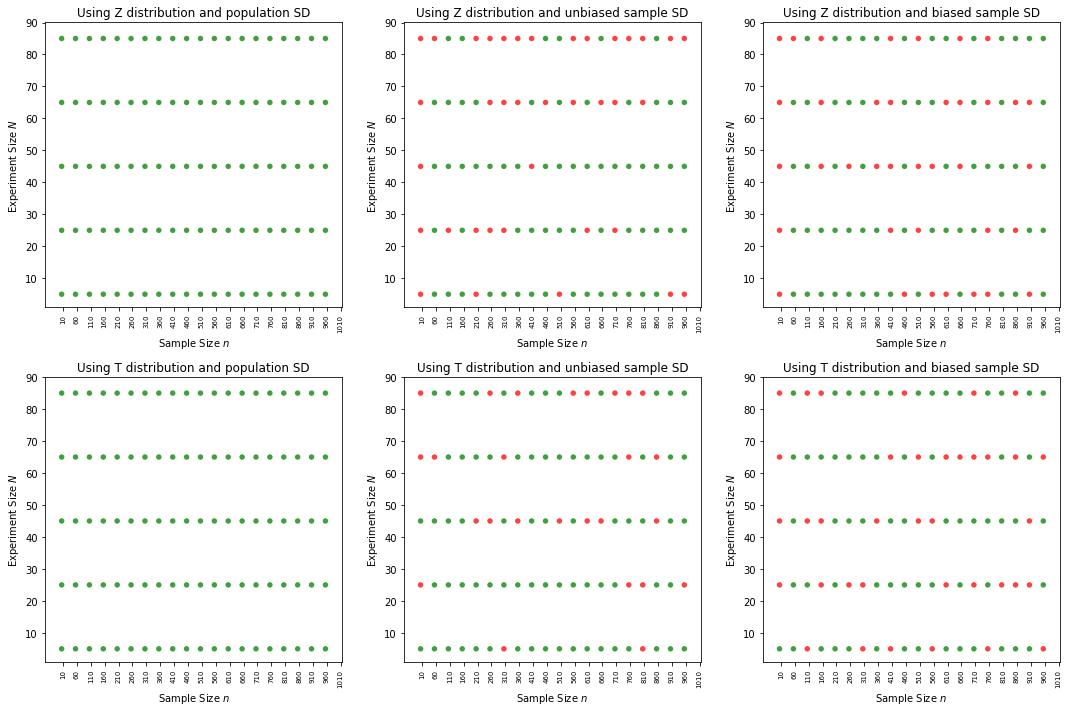Varying N,n, Sample without replacement¶

In :
from ci_helpers import plot_ci_accuracy_2, get_dist_label, get_mode_label

fig, axarr = plt.subplots(2,3, figsize=(15,10))

m , _ , s = get_metrics(population)
dist = 0
max_sample_size = int(T/4)  # 25% of total population
N_list = range(5,100,20)
n_list = range(5,max_sample_size,50)  # different sample sizes

for i in range(1,4): # 1,2,3 modes
for each_N in N_list:
for each_n in n_list:

dist=0  # z dist
ax = axarr[0,i-1]
accuracy_list = repeated_experiments_with_CI(population,m,s,[each_N],[each_n],i,dist,'b', replace=False)
plot_ci_accuracy_2(ax, accuracy_list)
ax.set_title('Using {} and {}'.format(get_dist_label(dist), get_mode_label(i)))

dist=1   # t dist
ax = axarr[1,i-1]
accuracy_list = repeated_experiments_with_CI(population,m,s,[each_N],[each_n],i,dist,'b', replace=False)
plot_ci_accuracy_2(ax, accuracy_list)
ax.set_title('Using {} and {}'.format(get_dist_label(dist), get_mode_label(i)))

plt.tight_layout()
plt.show()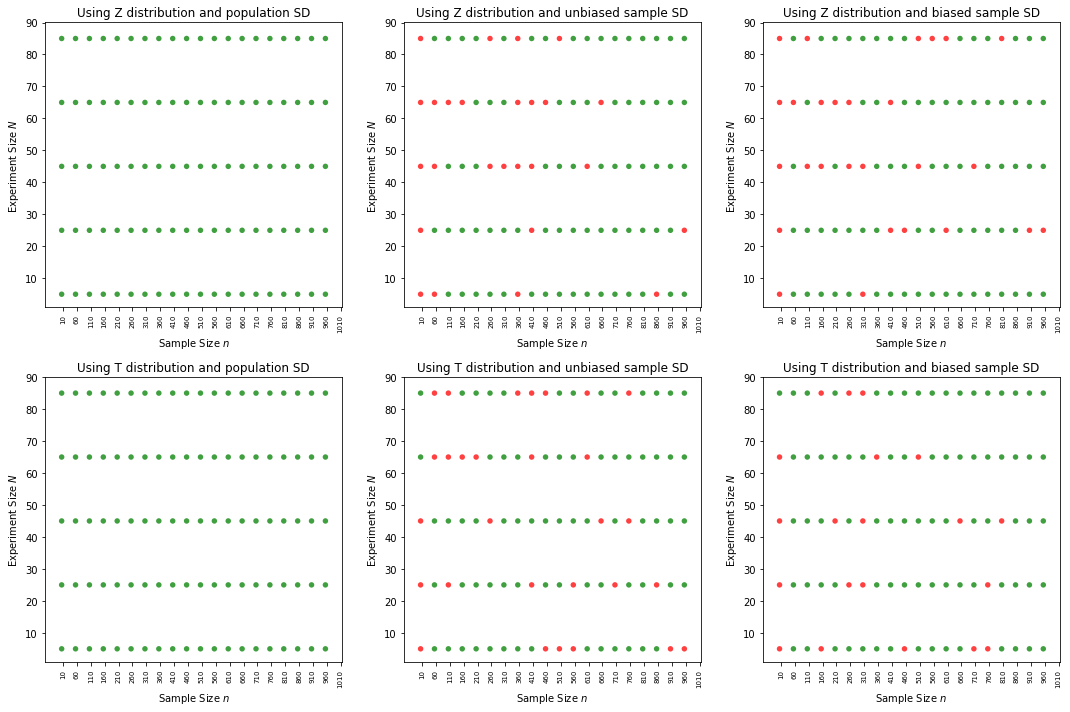Alternate faster methods¶

In :
from math import sqrt
from random import sample, choices

def repeated_experiments_with_CI_turbo(population,mu, sigma, N_list=[], n_list=[],  mode=1, dist=0, format='b', replace=True):
"""
population - raw population from which sample to be taken
mu, sigma - population parameters
C - 85% CI constant (for eg, 1.96 or 2.093 etc)
N_list - Experiment size or no of times experiments to be conducted per trial
n_list - Sample size or no of samples per experiment
Mode 1 - use population SD
Mode 2 - use unbiased sample SD
Mode 3 - usebiased sample SD
dist - 0 - use Z distribution
dist - 1 - use t distribution
"""
accuracy_list = []
for each_N in N_list:  # no of experiments
for each_n in n_list:   # sample size for each experiment
err_count = 0
for each_E in range(each_N): # for each experiment of experiment size
#                 Y_hat = sample(population, k=each_n)  # pick n samples
#                 Y_hat = choice(population, size=each_n, replace=replace)
if replace == True:
Y_hat = choices(population, k=each_n)
else:
Y_hat = sample(population, k=each_n)
Y_mean = sum(Y_hat)/len(Y_hat)

if dist == 0:
C = 1.96
elif dist == 1:
from scipy import stats
C = stats.t.ppf(1-0.025, each_n-1)  # t value varies depending on degrees of freedom  which is (no of samples - 1)

if mode == 1:
Y_sigma = sigma
CI_err = round(C*Y_sigma,4)  # if population SD, there is no sample size in denominator
elif mode == 2:
Y_variance = sum([(y - Y_mean)**2 for y in Y_hat])/(each_n-1)  # unbiased estimator
Y_sigma = round(sqrt(Y_variance), 4)
CI_err = round(C*(Y_sigma/sqrt(each_n)),4)
elif mode == 3:
Y_variance = sum([(y - Y_mean)**2 for y in Y_hat])/(each_n)  # biased estimator
Y_sigma = round(sqrt(Y_variance), 4)
CI_err = round(C*(Y_sigma/sqrt(each_n)),4)
else:
raise ValueError('Wrong mode')
low_err = Y_mean - CI_err
hig_err = Y_mean + CI_err
#print(CI_err,Y_mean,low_err,hig_err,mu)
if (hig_err <= mu) or (low_err >= mu):
err_count += 1
accuracy = round((1-err_count/each_N)*100,4)
success = 0 if accuracy < 95 else 1
if format == 'b':
accuracy_list.append((each_N, each_n, success))
else: # anything other than b
accuracy_list.append((each_N, each_n, accuracy))
return accuracy_list

With replacement¶

In :
from ci_helpers import plot_ci_accuracy_2, get_dist_label, get_mode_label

fig, axarr = plt.subplots(2,3, figsize=(15,10))

m , _ , s = get_metrics(population)
dist = 0
max_sample_size = int(T/4)  # 25% of total population
N_list = range(5,100,20)
n_list = range(5,max_sample_size,50)  # different sample sizes

for i in range(1,4): # 1,2,3 modes
for each_N in N_list:
for each_n in n_list:

dist=0  # z dist
ax = axarr[0,i-1]
accuracy_list = repeated_experiments_with_CI_turbo(population,m,s,[each_N],[each_n],i,dist,'b', replace=True)
plot_ci_accuracy_2(ax, accuracy_list)
ax.set_title('Using {} and {}'.format(get_dist_label(dist), get_mode_label(i)))

dist=1   # t dist
ax = axarr[1,i-1]
accuracy_list = repeated_experiments_with_CI_turbo(population,m,s,[each_N],[each_n],i,dist,'b', replace=True)
plot_ci_accuracy_2(ax, accuracy_list)
ax.set_title('Using {} and {}'.format(get_dist_label(dist), get_mode_label(i)))

plt.tight_layout()
plt.show()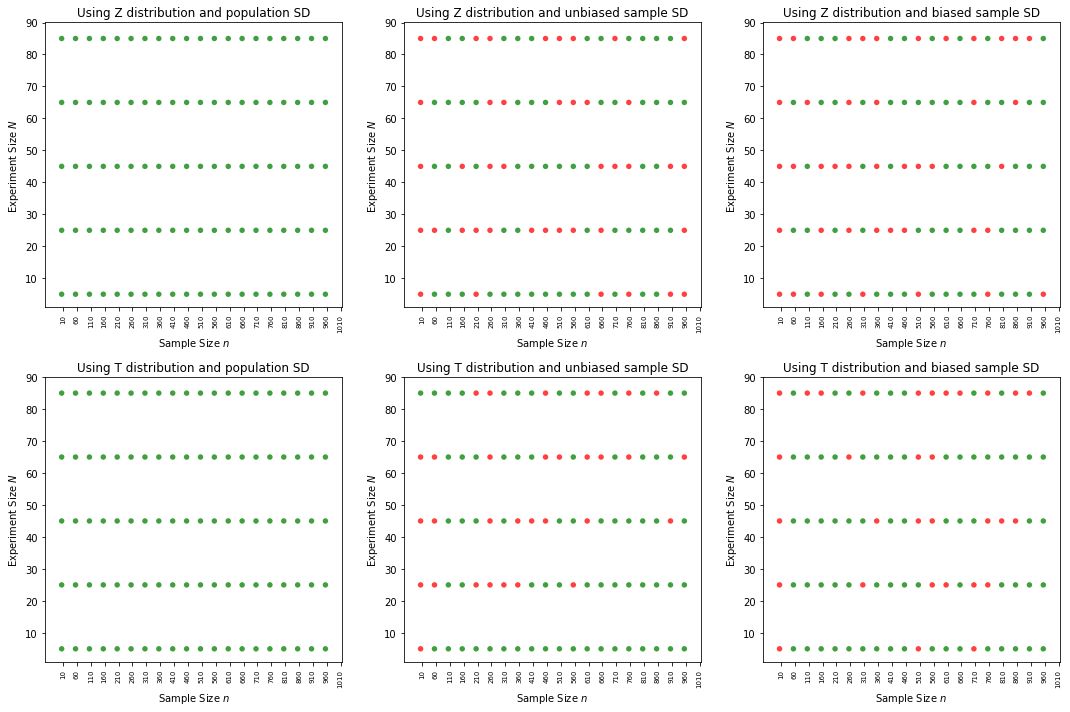Without replacement¶

In :
from ci_helpers import plot_ci_accuracy_2, get_dist_label, get_mode_label

fig, axarr = plt.subplots(2,3, figsize=(15,10))

m , _ , s = get_metrics(population)
dist = 0
max_sample_size = int(T/4)  # 25% of total population
N_list = range(5,100,20)
n_list = range(5,max_sample_size,50)  # different sample sizes

for i in range(1,4): # 1,2,3 modes
for each_N in N_list:
for each_n in n_list:

dist=0  # z dist
ax = axarr[0,i-1]
accuracy_list = repeated_experiments_with_CI_turbo(population,m,s,[each_N],[each_n],i,dist,'b', replace=False)
plot_ci_accuracy_2(ax, accuracy_list)
ax.set_title('Using {} and {}'.format(get_dist_label(dist), get_mode_label(i)))

dist=1   # t dist
ax = axarr[1,i-1]
accuracy_list = repeated_experiments_with_CI_turbo(population,m,s,[each_N],[each_n],i,dist,'b', replace=False)
plot_ci_accuracy_2(ax, accuracy_list)
ax.set_title('Using {} and {}'.format(get_dist_label(dist), get_mode_label(i)))

plt.tight_layout()
plt.show()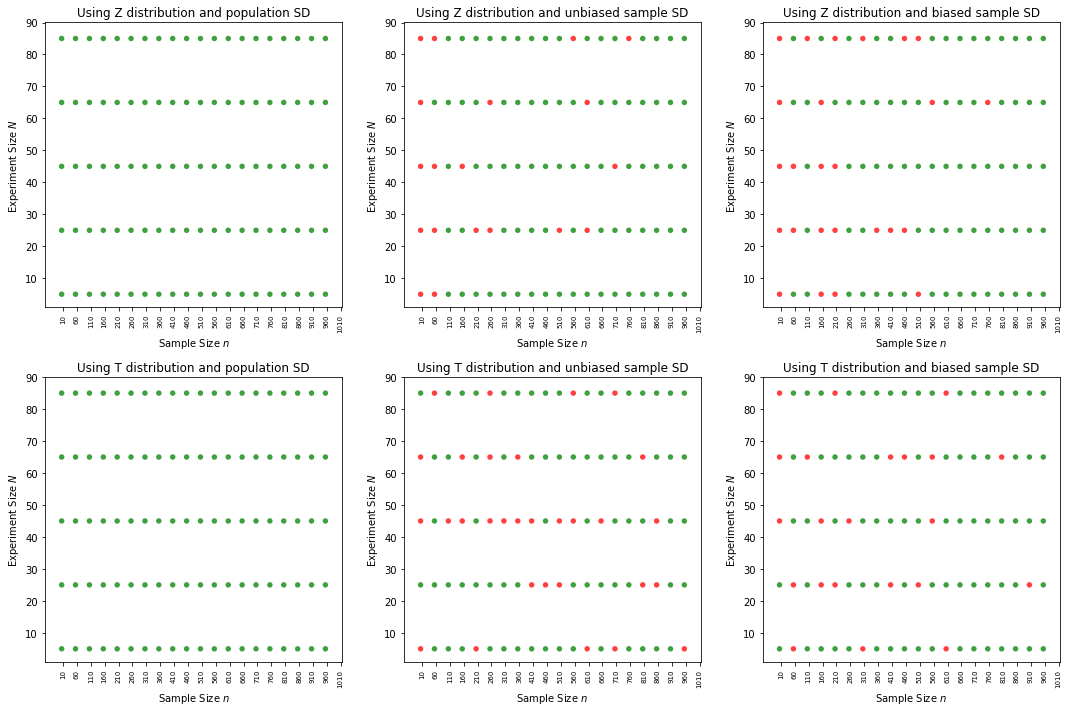T dist, Unbiased Sample SD for closer look why mixed results¶

Solution: Looks like Finite population correction was missing which was giving "without replacement" an edge, because variance was wider. With FPC, both are behaving bad(?!). But that should be understandable. You see, we use sample statistic SD, in place of population SD, so a performance reduction is expected. And also for most part, they are above 90% which is good enough..

In :
from ci_helpers import plot_ci_accuracy_2, get_dist_label, get_mode_label
from math import sqrt

def repeated_experiments_with_CI_madmax(population,mu, sigma, each_N, each_n,  mode=1, dist=0, format='b', replace=True):
"""
population - raw population from which sample to be taken
mu, sigma - population parameters
C - 85% CI constant (for eg, 1.96 or 2.093 etc)
N_list - Experiment size or no of times experiments to be conducted per trial
n_list - Sample size or no of samples per experiment
Mode 1 - use population SD
Mode 2 - use unbiased sample SD
Mode 3 - usebiased sample SD
dist - 0 - use Z distribution
dist - 1 - use t distribution
"""
accuracy_list = []
err_count = 0
T = len(population)
for each_E in range(each_N): # for each experiment of experiment size

if replace == True:
Y_hat = choices(population, k=each_n)  # choices by default samples with replacement
fpc = 1
else:
Y_hat = sample(population, k=each_n)   # sample by default samples without replacement
fpc = sqrt((T-each_n)/(T-1))
Y_mean = sum(Y_hat)/len(Y_hat)

if dist == 0:
C = 1.96
elif dist == 1:
from scipy import stats
C = stats.t.ppf(1-0.025, each_n-1)  # t value varies depending on degrees of freedom  which is (no of samples - 1)

if mode == 1:
Y_sigma = sigma*fpc          # fpc affects depending on with or without replacement
CI_err = round(C*Y_sigma,4)  # if population SD, there is no sample size in denominator
elif mode == 2:
Y_variance = sum([(y - Y_mean)**2 for y in Y_hat])/(each_n-1)  # unbiased estimator
Y_sigma = round(sqrt(Y_variance), 4)
Y_sigma = Y_sigma*fpc
CI_err = round(C*(Y_sigma/sqrt(each_n)),4)
elif mode == 3:
Y_variance = sum([(y - Y_mean)**2 for y in Y_hat])/(each_n)  # biased estimator
Y_sigma = round(sqrt(Y_variance), 4)
Y_sigma = Y_sigma*fpc
CI_err = round(C*(Y_sigma/sqrt(each_n)),4)
else:
raise ValueError('Wrong mode')
low_err = Y_mean - CI_err
hig_err = Y_mean + CI_err
#print(CI_err,Y_mean,low_err,hig_err,mu)
if (hig_err <= mu) or (low_err >= mu):
err_count += 1
accuracy = round((1-err_count/each_N)*100,4)
success = 0 if accuracy < 95 else 1
if format == 'b':
accuracy_list.append((each_N, each_n, success))
else: # anything other than b
accuracy_list.append((each_N, each_n, accuracy))
return accuracy_list

def plot_ci_accuracy_3(ax, accuracy_list):
# for continous format of accuracy list.. gradient coloring
y,x,z=zip(*accuracy_list)
points = ax.scatter(x, y, c=z, cmap='RdYlGn')

xmin = 10  # starting with sample size 10
xmax = ax.get_xlim()
from math import ceil,floor
xminint = floor(xmin)
xmaxint = ceil(xmax)
xint = range(xminint, xmaxint, 50)
ax.set_xticks(xint)
ax.xaxis.set_tick_params(labelsize=7)
for tick in ax.get_xticklabels():
tick.set_rotation(90)

ax.set_ylabel('Experiment Size $N$')
ax.set_xlabel('Sample Size $n$')

return points

import matplotlib.pyplot as plt
fig, axarr = plt.subplots(2,2, figsize=(10,10))
m , _ , s = get_metrics(population)
dist = 0
max_sample_size = int(T/4)  # 25% of total population
N_list = range(10,500,20)
n_list = range(10,max_sample_size,50)  # different sample sizes
acc_list_1 = []
acc_list_2 = []

for each_N in N_list:
for each_n in n_list:
i = 2
dist=1   # t dist

ax = axarr[0,0]
plot_ci_accuracy_2(ax, accuracy_list)
ax.set_title('Sampling With Replacement\nUsing {} and {}'.format(get_dist_label(dist), get_mode_label(i)))

ax = axarr[0,1]
plot_ci_accuracy_2(ax, accuracy_list)
ax.set_title('Sampling Without Replacement\nUsing {} and {}'.format(get_dist_label(dist), get_mode_label(i)))

acc_list_1.append(accuracy)

acc_list_2.append(accuracy)

# plotting individual CIs in color
vmin = 80
vmax = 100
vstep = 5

ax = axarr[1,0]
points = plot_ci_accuracy_3(ax, acc_list_1)
# points.set_clim(vmin,vmax)
fig.colorbar(points, ax=ax)#, ticks=list(range(vmin,vmax+vstep,vstep)))

ax = axarr[1,1]
points = plot_ci_accuracy_3(ax, acc_list_2)
# points.set_clim(vmin,vmax)
fig.colorbar(points, ax=ax)#, ticks=list(range(vmin,vmax+vstep,vstep)))

plt.tight_layout()
plt.show()